# Capacitive Touch Pad with Raspberry Pi

Published  June 4, 2016   0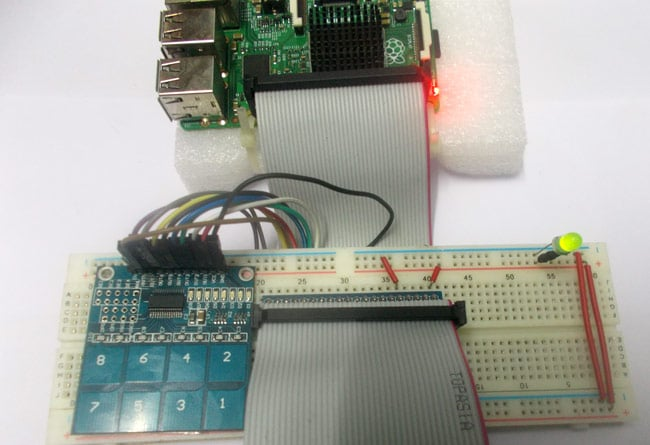Raspberry Pi is an ARM architecture processor based board designed for electronic engineers and hobbyists. The PI is one of most trusted project development platforms out there now. With higher processor speed and 1 GB RAM, the PI can be used for many high profile projects like Image processing and Internet of Things.

For doing any of high profile projects, one need to understand the basic functions of PI. We will be covering all the basic functionalities of Raspberry Pi in these tutorials. In each tutorial we will discuss one of functions of PI. By the end of this Raspberry Pi Tutorial Series, you will be able to do high profile projects by yourself. Go through below tutorials:

In this tutorial, we will Interface a Capacitive Touchpad to Raspberry Pi. Capacitive Touchpad has 8 keys from 1 to 8. These keys are not exactly keys, they are Touch Sensitive Pads placed on the PCB. When we touch one of the pads, the pads experience the change of capacitance on its surface. This change is captured by the control unit and control unit, as a response, pulls a corresponding pin high at the output side.

We will attach this Capacitive Touchpad Sensor Module to the Raspberry Pi, to use it as input device for the PI.

We will discuss a bit about Raspberry Pi GPIO Pins before going any further.

### GPIO Pins: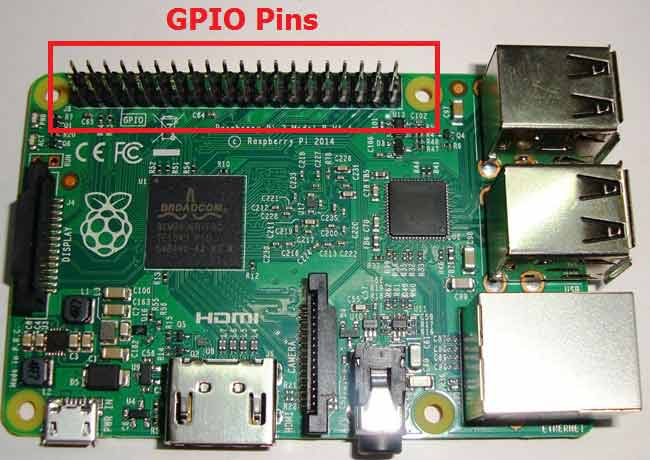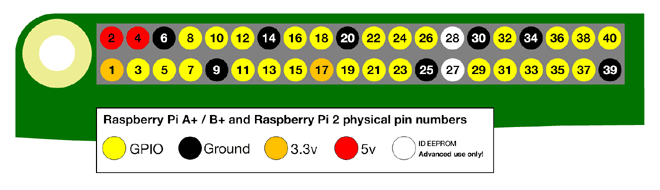As shown in above figure, there are 40output pins for the PI. But when you look at the second figure below, you can see not all 40 pin out can be programmed to our use. These are only 26 GPIO pins which can be programmed. These pins go from GPIO2 to GPIO27.

These 26 GPIO pins can be programmed as per need. Some of these pins also perform some special functions, we will discuss about that later. With special GPIO put aside, we have 17 GPIO remaining (Light green Color).

Each of these 17 GPIO pins can deliver a maximum of 15mA current. And the sum of currents from all GPIO cannot exceed 50mA. So we can draw a maximum of 3mA in average from each of these GPIO pins. So one should not tamper with these things unless you know what you are doing.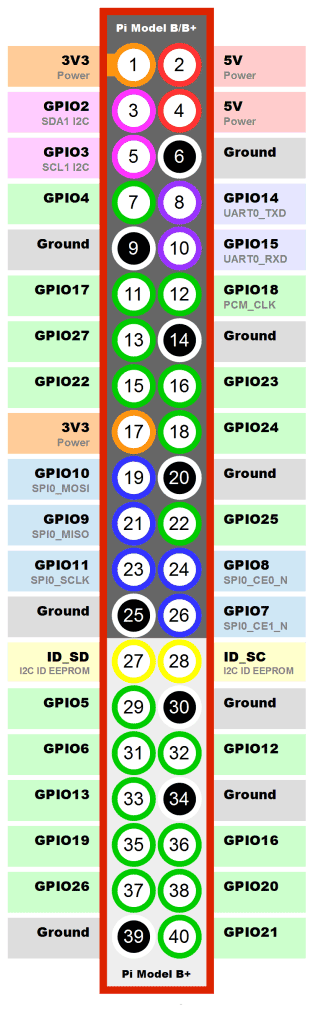Now another important thing here is that, PI logic control is of +3.3v, so you cannot give more than +3.3V logic to GPIO pin of PI. If you give +5V to any GPIO pin of PI, the board gets damaged. So we need to power the Capacitive Touchpad by +3.3V, for getting proper logic outputs for PI.

### Components Required:

Here we are using Raspberry Pi 2 Model B with Raspbian Jessie OS. All the basic Hardware and Software requirements are previously discussed, you can look it up in the Raspberry Pi Introduction, other than that we need:

• Connecting pins

### Circuit Diagram: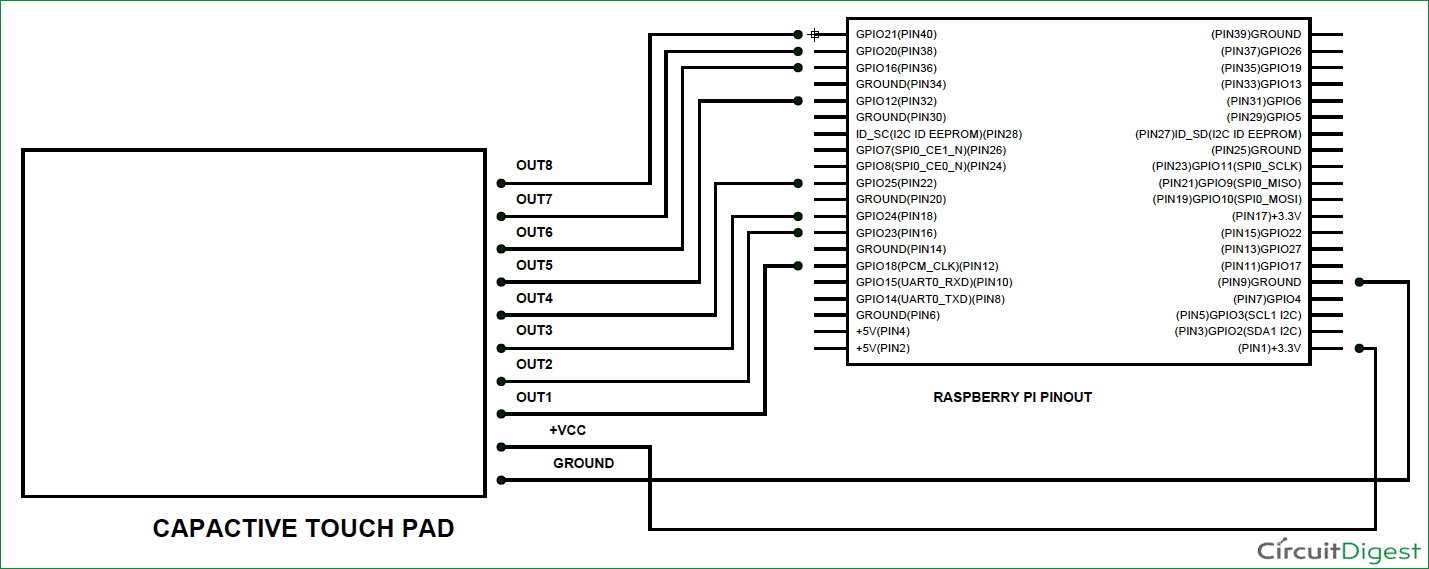The connections, which are done for Capacitive Touchpad Interfacing, are shown in the circuit diagram above.

### Working and Programming Explanation:

Once everything is connected as per the circuit diagram, we can turn ON the PI to write the program in PYHTON.

We will talk about few commands which we are going to use in PYHTON program,

We are going to import GPIO file from library, below function enables us to program GPIO pins of PI. We are also renaming “GPIO” to “IO”, so in the program whenever we want to refer to GPIO pins we will use the word ‘IO’.

`import RPi.GPIO as IO`

Sometimes, when the GPIO pins, which we are trying to use, might be doing some other functions. In that case, we will receive warnings while executing the program. Below command tells the PI to ignore the warnings and proceed with the program.

`IO.setwarnings(False)`

We can refer the GPIO pins of PI, either by pin number on board or by their function number. Like ‘PIN 29’ on the board is ‘GPIO5’. So we tell here either we are going to represent the pin here by ‘29’ or ‘5’.

`IO.setmode (IO.BCM)`

We are setting 8 pins as input pins. We will detect 8 key outputs from Capacitive Touchpad.

```IO.setup(21,IO.IN)
IO.setup(20,IO.IN)
IO.setup(16,IO.IN)
IO.setup(12,IO.IN)
IO.setup(25,IO.IN)
IO.setup(24,IO.IN)
IO.setup(23,IO.IN)
IO.setup(18,IO.IN)```

In case the condition in the braces is true, the statements inside the loop will be executed once. So if the GPIO pin 21 goes high, then the statements inside the IF loop will be executed once. If the GPIO pin 21 does not goes high, then the statements inside the IF loop will not be executed.

`if (IO.input(21) == True):`

Below command is used as forever loop, with this command the statements inside this loop will be executed continuously.

`While 1:`

Once we write the below program in PYTHON and execute it we are ready to go. When the pad is touched, the module pulls up the corresponding pin and this trigger is detected by the PI. After the detection, the PI prints the appropriate key on the screen.

Hence we have Interfaced Capacitive Touchpad to PI.

Code

import RPi.GPIO as IO                # calling for header file for GPIO’s of PI
import time                                  # calling for time to provide delays in program
IO.setwarnings(False)                 # do not show any warnings
IO.setmode (IO.BCM)                # programming the GPIO by BCM pin numbers. (like PIN29 as‘GPIO5’)
IO.setup(21,IO.IN)                     # initialize GPIO Pins as an Input.
IO.setup(20,IO.IN)
IO.setup(16,IO.IN)
IO.setup(12,IO.IN)
IO.setup(25,IO.IN)
IO.setup(24,IO.IN)
IO.setup(23,IO.IN)
IO.setup(18,IO.IN)

while 1:
if (IO.input(21) == True):
time.sleep(0.001)
if (IO.input(21) == True):
print ( 8)                                # if PIN21 is high print’8’
if (IO.input(20) == True):
time.sleep(0.001)
if (IO.input(20) == True):
print ( 7)                                # if PIN20 is high print’7’
if (IO.input(16) == True):
time.sleep(0.001)
if (IO.input(16) == True):
print ( 6)                                # if PIN16 is high print’6’
if (IO.input(12) == True):
time.sleep(0.001)
if (IO.input(12) == True):
print ( 5)                                 # if PIN12 is high print’5’
if (IO.input(25) == True):
time.sleep(0.001)
if (IO.input(25) == True):
print ( 4)                                # if PIN25 is high print’4’
if (IO.input(24) == True):
time.sleep(0.001)
if (IO.input(24) == True):
print ( 3)                                # if PIN24 is high print’3’
if (IO.input(23) == True):
time.sleep(0.001)
if (IO.input(23) == True):
print ( 2)                                # if PIN23 is high print’2’
if (IO.input(18) == True):
time.sleep(0.001)
if (IO.input(18) == True):
print ( 1)                                # if PIN18 is high print’1’
time.sleep(0.2)                              # wait for 200ms

Video

Tags